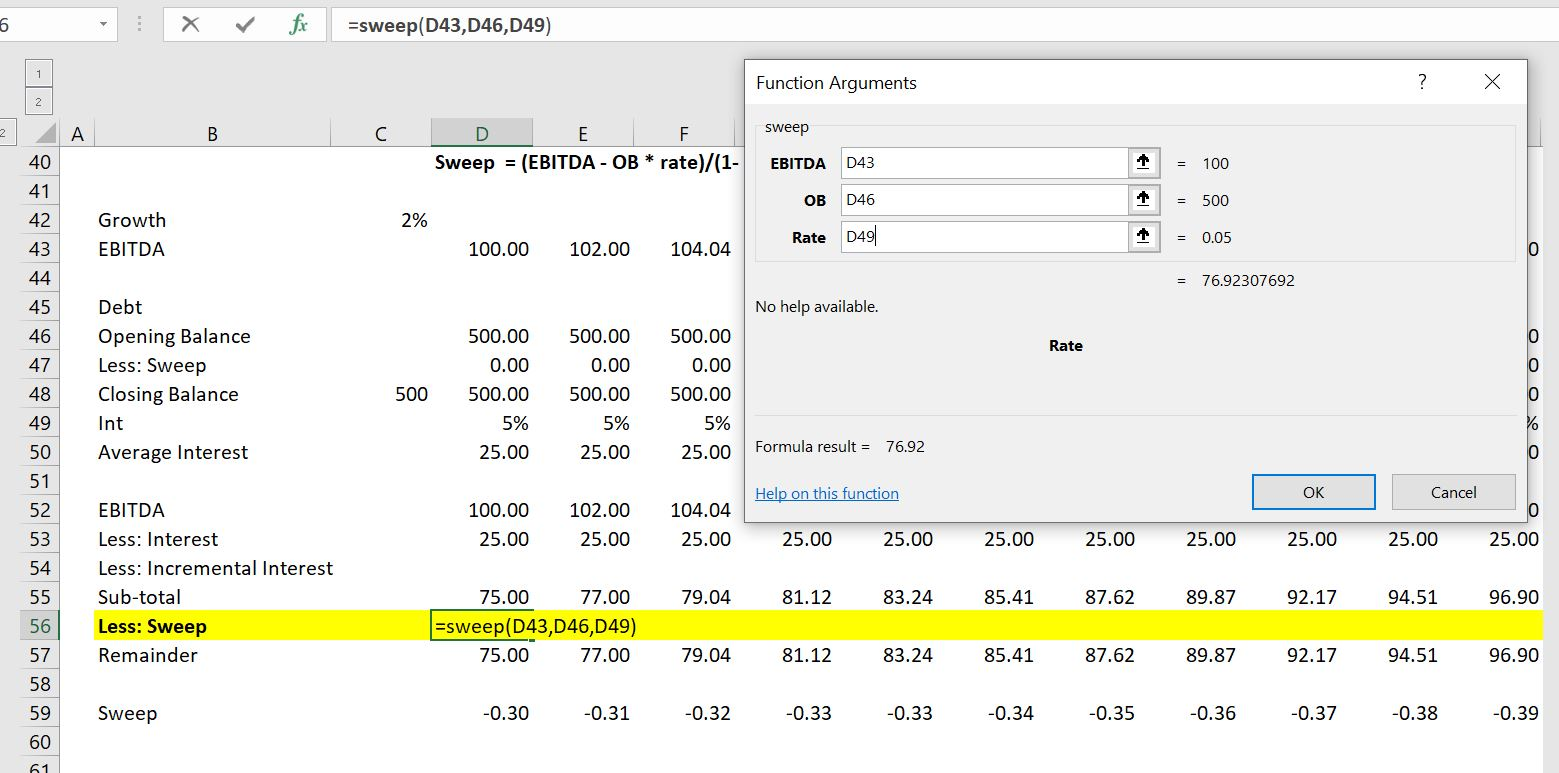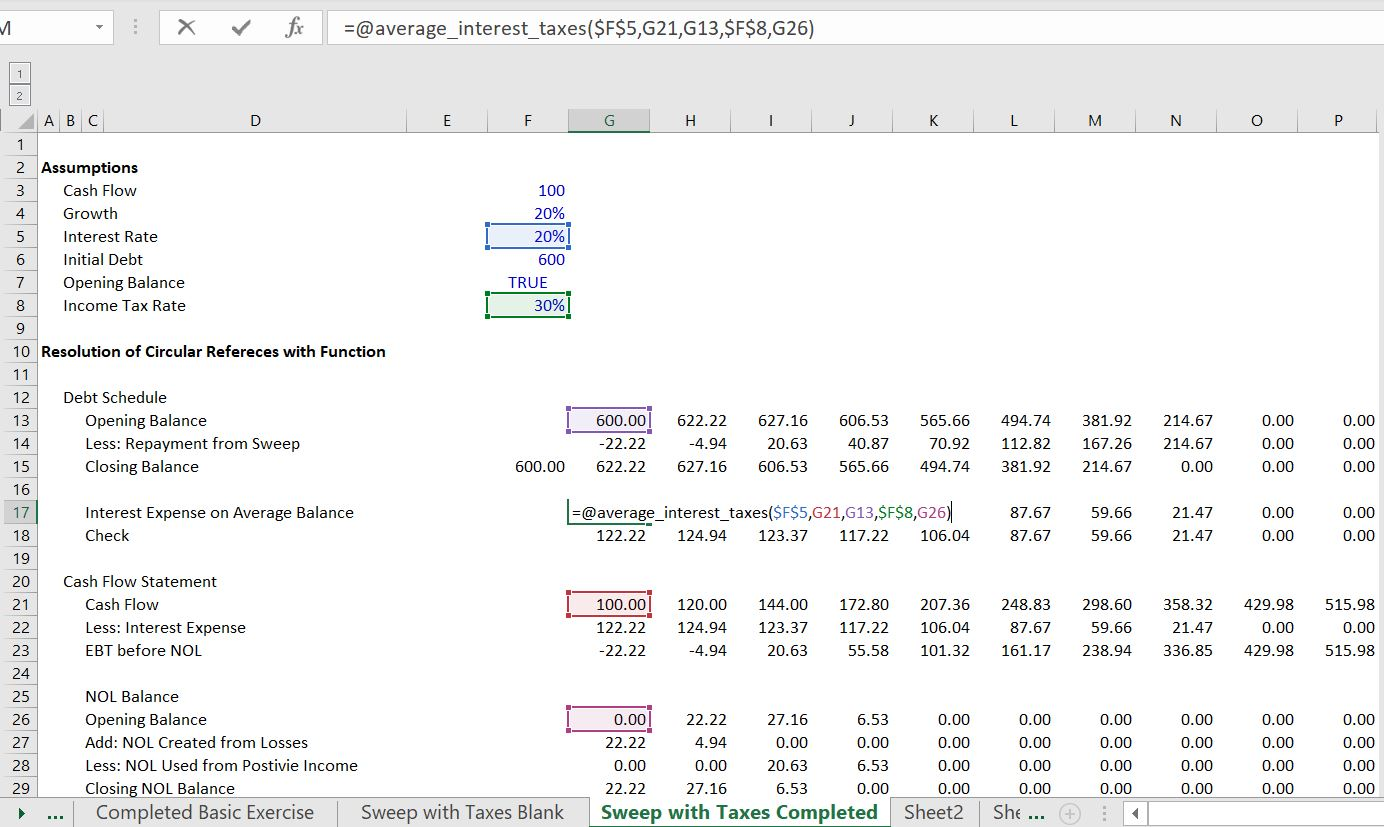# Circular References in Corporate Finance

I explain how you can resolve a couple of circular references that arise in corporate models on this page. The files on this page show how to use a copy and paste macro that breaks a model and a user defined function method that resolves the circular reference smoothly. I first deal with the case of a cash flow sweep in an LBO or structured finance model and then move to the case of a more classic corporate model. The most classic circular reference comes from interest expense and interest income that is affected by financing over the course of the year. In an annual model interest can be computed on the average balance. If you don’t make this assumption you are implicitly assuming that all activity for the corporation comes at the very end of the year. Developing a user defined function is not that difficult to resolve the circular reference because no loop is required. The video and files below illustrate how to solve this circular reference.

## Don’t Believe People who Give you B.S. About Circular References

My good friend Karnen sent be an article about circular references written by some professor.  This man claimed that you can somehow get around circular references.  What total rubbish.  Circular reference do arise in all sorts of financial models.  For corporate models, circular references are driven by the fact that models may be annual or even quarterly, but the interest expense is not paid to the lender in the same increments as the model. Where the interest expense is not computed from the opening balance of debt, interest expense and income affects cash flow and the cash flow affects financing and the amount of the loan. If the interest expense is determined in part from the closing balance of the loan, a circular reference arises. Unless you make a monthly model there will be a circular reference because of the timing assumption in a financial model.

There are various ways to deal with circular references in models. The first method is to use the iteration button in excel. But this can cause many problems that I have documented elsewhere — no goal seek; problems with undo, model instability etc. Another method is the copy and paste macro.  In corporate models, the copy and paste method is very clumsy and prevents you from using many very useful tools in excel. In this page, I discuss how you can solve the problem with a user defined function.  With a UDF, you can replace the interest expense in the model with a function.  In writing the function you write down the equations of the model in parallel.

## Very Simple Case

In the example below I illustrate a case where a loan is repaid with a cash flow sweep.  The interest is computed on the average debt and not the initial debt. As the interest expense is a deduction for cash flow calculation.  So the interest drives the cash flow and the cash flow drives debt which drives interest.  The first screenshot below illustrates the interest calculation.The UDF can resolve this circular reference in a very easy way.  You just create a little program that defines the sweep as an algebraic formula which is shown first.  Alternatively, the second function demonstrates how to work through the same equations in parallel.  You begin by writing an equation for the sweep and then work backwards.

```.

Function sweep(EBITDA, OB, Rate)

sweep = (EBITDA - OB * Rate) / (1 - Rate / 2)

End Function

Function average_interest(interest_rate, cash_flow, opening_debt_balance)

For Iteration = 1 To 30
last_sweep = sweep

average_interest = ((opening_debt_balance + closing_debt_balance) / 2) * interest_rate

sweep = WorksheetFunction.Min(cash_flow - average_interest, opening_debt_balance)

closing_debt_balance = opening_debt_balance - sweep

Next Iteration

converge = Abs(sweep - last_sweep)

If converge < 0.000001 Then Exit Function

End Function
.```

The screenshot below illustrates how to implement the UDF.  Note that you can find the inputs with either the Shift and F3 short-cut or with the fx thing in the formula bar.## A More Complex Case with Taxes and NOL

With taxes, the taxes affect the cash flow, but the taxes are affected by the interest expense.  Here, the tax calculations must be part of the UDF.  The UDF function is demonstrated below the screenshot.  I hope that you can see that the formulas are just the same as the formulas in the spreadsheet with some convergence routines added.```Function average_interest_taxes(interest_rate, cash_flow, opening_debt_balance, tax_rate, opening_NOL_Balance)

For Iteration = 1 To 30
last_sweep = sweep

average_interest_taxes = ((opening_debt_balance + closing_debt_balance) / 2) * interest_rate

taxable_income = cash_flow - average_interest_taxes
NOL_created = WorksheetFunction.Max(0, 0 - taxable_income)
NOL_used = WorksheetFunction.Min(opening_NOL_Balance, WorksheetFunction.Max(0, taxable_income))
closing_NOL_Balance = opening_NOL_Balance + NOL_created - NOL_used
adjusted_taxable_income = taxable_income + NOL_created - NOL_used

sweep = WorksheetFunction.Min(cash_flow - average_interest_taxes - taxes_paid, opening_debt_balance)

closing_debt_balance = opening_debt_balance - sweep

Next Iteration

converge = Abs(sweep - last_sweep)

If converge < 0.000001 Then Exit Function

End Function

```

## Circular References in Classic Corporate Models

Circular references with a similar interest expense problem are common in corporate models.  A common approach is to just use the opening balance of the short-term debt and other investments when the interest rates are low.  But if the interest expense and interest income is computed on some kind of average daily basis and if you are in a place where interest expense and interest income rates are high, you can take a few minutes and add a similar UDF to your models.

## Videos on Mechanics of Resolving Circular Reference in Corporate Models

Circular Reference Exercise 3 – Corporate Modelxlsm.xlsm
Exercise 10 – Target Capital Structure with Function.xlsm